### Vectors and their Operations: Dot product

### The dot product and its properties

The dot product, also called the scalar product, is an operation that takes two vectors and returns a scalar. The dot product of vectors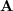and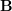, denoted as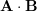and read “dot” is defined as:

(2.14)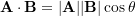where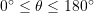is the angle between the two vectors (Fig. 2.24)

From the definition, it is obvious that the result of the dot product is a scalar. The dot product has three properties as follows:

1. Commutativity: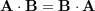2. Associativity (scalar multiplication):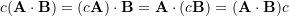3. Distributivity: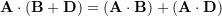The proofs of the first two properties are by direct use of the dot product definition (Eq. 2.14). The proof for the third property is by expanding the right hand side of the equation using CVN and using the properties explained below.

##### Other properties of the dot product
1. Dot product of a vector by itself gives its squared magnitude: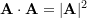.
2. Dot product of two perpendicular vectors is zero: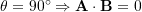.
3. Dot product by the zero vector is zero: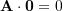.

These properties can be easily proved using Eq. 2.14.

### Formulation of the dot product using CVN

Letandbe two vectors with their scalar components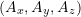and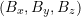. Using CVN, we can write: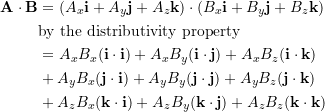The dot product of the unit vectors, by the dot product properties, are: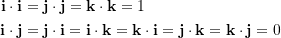Therefore,

(2.15)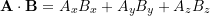This result expresses that the dot product of two vectors written in their CVN can be obtained by multiplying their corresponding scalar components and summing over these products algebraically. Equation 2.15 indicates that calculating the dot product (Eq. 2.14) does not need the magnitudes of two vectors and the angle between them, if the vectors are expressed in CVN.

### Application of the dot product: finding the angle between two vectors

The dot product can be used to find the angle formed between two vectors or two intersecting lines. This is helpful particularly when solving problems in three dimensions. The angle between two vectors is obtained by solving Eq. 2.14 for the angle term:

(2.16)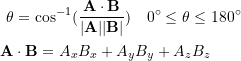The above equation can be manipulated as:

(2.17)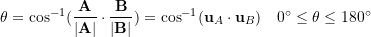in which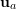and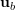are the unit vectors ofandrespectively. This result naturally states that the angle between two vectors only depends on their directions and not on their magnitudes.

### Application of the dot product: orthogonal projection of a vector

In many problems, we need to resolve a vector on a particular line or lines in the space. To be more precise, the component of a vector along a particular direction or axis is to be found. Decomposing a vector onto the Cartesian axes is already demonstrated. In this section, we explain decomposing a vector on a general line in space using the dot product. Using the dot product makes the calculation easier specially in three dimensions.

Consider a non-zero vectorin the three dimensional space and a lineintersecting the tail of the vector at a point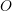(Fig. 2.25a). A unit vectoris associated with lineto assign a direction to the line. In other words, The positive direction of the line is determined by. As demonstrated in Fig. 2.25b, the vectorcan be written as,

(2.18)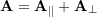where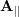is parallel to, and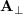is perpendicular to. The symbolsand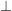denote being parallel and perpendicular respectively.

The vectoris referred to as the orthogonal projection (or projection) ofonto the lineor along the direction of. We denoteas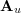to indicate thatis a projection along the direction of.

To obtain, it suffices to note that the vectors,andform a right-angle triangle (Fig. 2.25b). Therefore by the Pythagorean’s theorem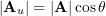. This inspires us to use Eq. 2.14 and write,

(2.19)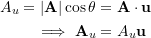It should be noted that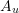​ is the scalar component of​ resolved along the direction of​. Using the dot product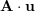​ to calculate​ may result in a negative scalar if the angle between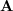and​ are larger than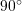​. In such a case, the direction of​ is in the opposite direction of​.

The following interactive tool illustrates the orthogonal projection of a vectoron the direction defined by a unit vector.

The perpendicular component ofcan be then obtained by writing,

(2.20)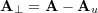The magnitude of the perpendicular component can be calculated either by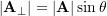or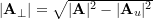.

In practice,andcan be readily used if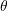in known, otherwise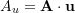,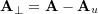, andcan be utilized if the components of the vectors in CVN are known.

As a special case, orthogonal projection is used to find the scalar components of a vector,in a Cartesian frame. This is done by writing:

(2.21)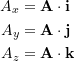### Videos

Dot Product:

Angle Between Vectors:

Orthogonal Projections: